# Tutorial: Antenna Gain and Directivity

By Jairam Sankar

94066

RF antennas or aerials do not radiate equally in all directions. It is found that any realisable RF antenna design will radiate more in some directions than others. The actual pattern is dependent upon the type of antenna design, its size, the environment and a variety of other factors. This directional pattern can be used to ensure that the power radiated is focused in the desired directions.

It is normal to refer to the directional patterns and gain in terms of the transmitted signal. It is often easier to visualise the RF antenna in terms of its radiated power, however, the antenna performs in an exactly equivalent manner for the reception, having identical figures and specifications.

## Directivity

The directivity of an antenna is the ratio of the maximum power density P(θ,φ)max to its average value over a sphere as observed in the far field of an antenna.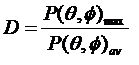It is a dimensionless ratio ≥ 1. The average power density over a sphere is given by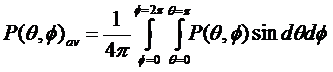ORSo, the directivity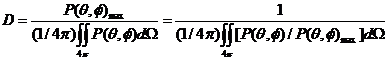Or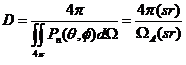The smaller the beam area ΩA, the larger the directivity D. In decibels,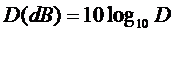The numerical value of D always lies between 1 and ∞. The idealized isotropic antenna radiates equally in all the directions, so its beam area ΩA = 4π sr.

Its directivity is :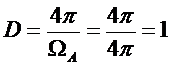This is the lowest possible directivity (D = 1). All actual antennas have directivities greater than 1 (D > 1).

## Antenna Gain

In electromagnetics, an antenna’s power gain or simply gain is a key performance number which combines the antenna’s directivity and electrical efficiency. As a transmitting antenna, the gain describes how well the antenna converts input power into radio waves headed in a specified direction. As a receiving antenna, the gain describes how well the antenna converts radio waves arriving from a specified direction into electrical power. When no direction is specified, “gain” is understood to refer to the peak value of the gain. A plot of the gain as a function of direction is called the radiation pattern.

Gain is related to directivity with antenna efficiency factor as:k or η: antenna efficiency factor (0 ≤ k ≤ 1), dimensionless. If k or η = 1, i.e. for a lossless antenna, .In practice, gain is always less than the directivity D.

Gain can be of following types:

Power Gain (Gp)
Directive Gain (Gd)

1. Power Gain (Gp): It is the ratio of radiation intensity in a given direction to the average total input power.Total input power PT = Pr + Pl

Pl: Ohmic losses in the antenna

2. Directive Gain (Gd): It is the ratio of radiation intensity in a particular direction to the average radiated power.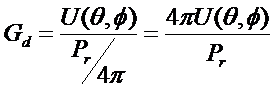Gd does not depend upon the power input to the antenna & its ohmic losses.

The maximum value of directive gain is the directivity D of the antenna.

Also,η: Efficiency factor which lies between 0 to 1

The author is a final year B.Tech ECE student at RSET,  Cochin

#### The article was first published on 22 July 2016 and was updated on 17 April 2019.

1.Nikhil

Thanks
It is very helpfull

2.Nitin

fundamentals nicely explained

3.Gang Lv

Good, I search the net for the content of attenna gain. But only this one is the best tutorial material and it is very helpful, I think.

•EFY Team

Thank you for your feedback.